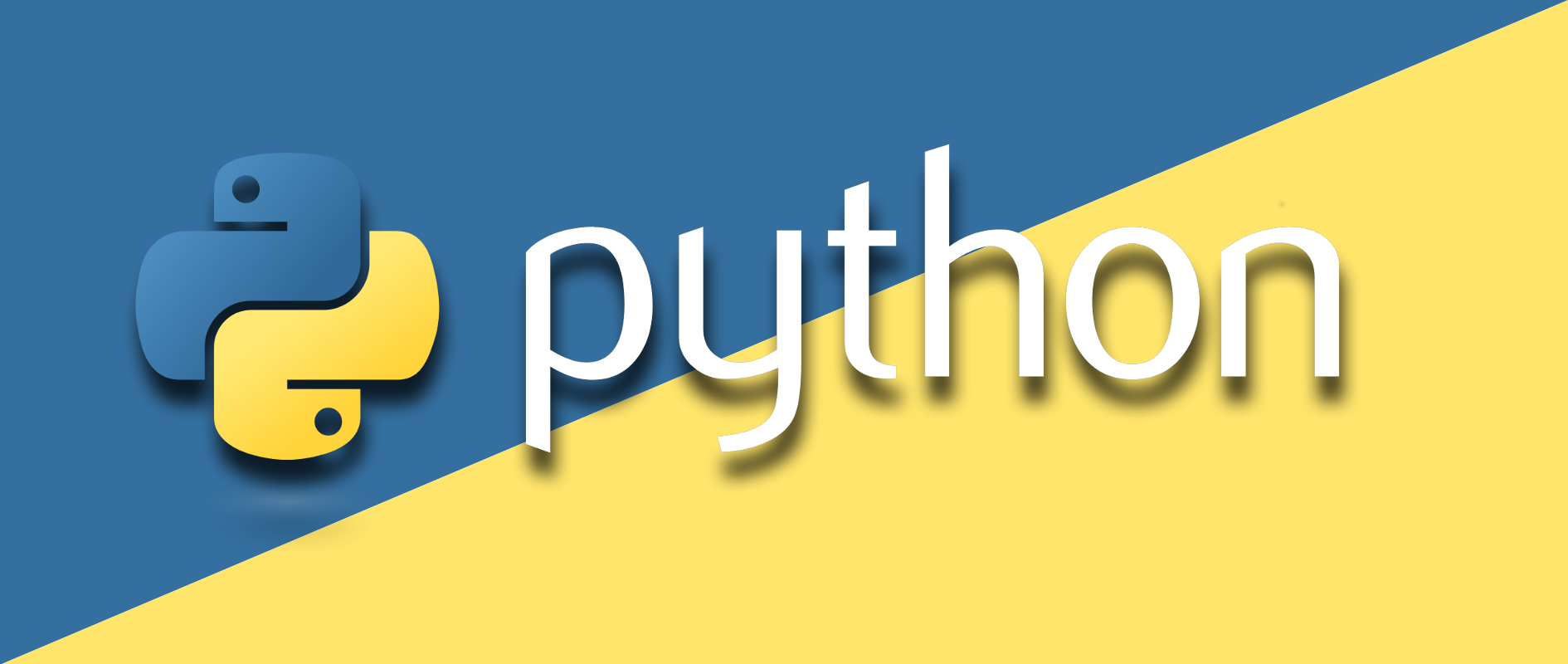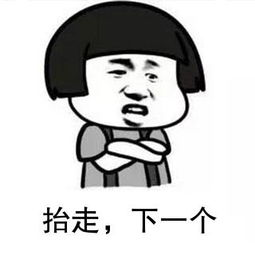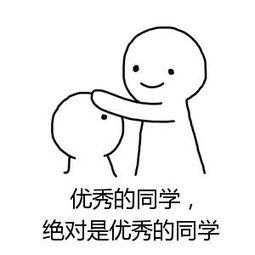# 小白学 Python（20）：迭代器基础## 迭代器

• 可以记住遍历的位置的对象。
• 迭代器从集合的第一个元素开始访问，直到所有的元素访问结束。
• 迭代器只能向前不能后退。

from collections.abc import Iterable

print(isinstance('geekdigging', Iterable))
print(isinstance([], Iterable))
print(isinstance([], Iterable))
print(isinstance({x for x in range(5)}, Iterable))
print(isinstance(123, Iterable))


True
True
True
True
False


D:/Development/Projects/python-learning/base-iter/Demo.py:1: DeprecationWarning: Using or importing the ABCs from 'collections' instead of from 'collections.abc' is deprecated, and in 3.8 it will stop working
from collections import Iterablelist1 = [1, 2, 3, 4]
next(list1)


Traceback (most recent call last):
File "D:/Development/Projects/python-learning/base-iter/Demo.py", line 12, in <module>
next(list1)
TypeError: 'list' object is not an iteratorlist1 = iter(list1)
print(type(list1))


<class 'list_iterator'>


print(next(list1))
print(next(list1))
print(next(list1))
print(next(list1))


1
2
3
4print(next(list1))


Traceback (most recent call last):
File "D:/Development/Projects/python-learning/base-iter/Demo.py", line 23, in <module>
print(next(list1))
StopIteration


set1 = {1, 2, 3, 4, 5}
set1 = iter(set1)
print(next(set1))
print(next(set1))
print(next(set1))
print(next(set1))
print(next(set1))## 示例代码

posted @ 2019-11-13 08:44  极客挖掘机  阅读(228)  评论(0编辑  收藏